# Altova UModel 2020 Enterprise Edition

 How to Model... > Diagrams Creating Diagrams Scroll Home Prev Top Next More

Diagrams represent visually how modeling elements interact, what is their structure, dependencies, hierarchy, and so on. Diagrams must belong to a package in the project, and therefore must be created under an existing package in the Model Tree window. You can move diagrams from one package to another at any time, by dragging them into a destination package.

To create a new diagram:

1.Right-click a package in the Model Tree window.

2.Select New Diagram | <Diagram Kind>.

You can also create a new diagram from the Diagram Tree window, as follows:

1.Right-click the root node ("Diagrams") in the Diagram Tree window.

2.Select a package where the diagram should belong, and click OK.

When the diagram window is active, the toolbars displays only modeling elements applicable to the current diagram kind. The diagram kind is displayed in the Properties window after you click an empty area of the diagram. In addition to this, the following icons depict the diagram kind.

Icon

Description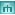Activity Diagram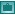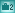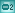BPMN 2 Choreography Diagram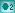BPMN 2 Collaboration Diagram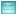Class Diagram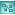Communication Diagram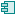Component Diagram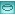Composite Structure Diagram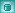Database Diagram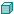Deployment Diagram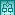Interaction Overview Diagram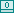Object Diagram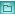Package Diagram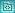Profile Diagram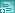Protocol State Machine Diagram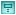Sequence Diagram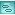State Machine Diagram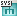SysML diagrams (9 diagram types)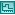Timing Diagram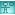Use Case Diagram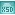XML Schema Diagram